# Low Pass RC Circuit Response to a Square Wave

The Laplace transform is used to study the response of RC circuits to a square wave input; numerical examples with graphs of volatges are presented.
An online calculator and grapher on low pass RC circuit response to a square wave is also included.



## Problem with Solution

Find and graph the voltages across the capacitor $C$ and the resistor $R$ and the current $i$ as functions of time in the ciruit below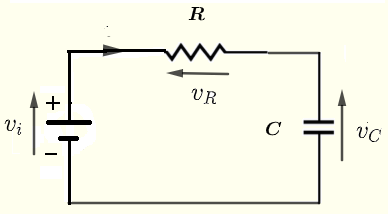Fig.1 - Low Pass RC circuit given that the input voltage is $v_i(t)$ is a square wave as shown in the graph below.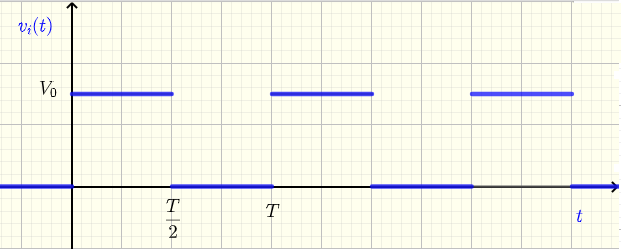Fig.2 - Square Wave as Input to the RC Circuit Solution to the Problem
The equation, in the $s$ domain , relating the voltage $V_C(s)$ across the capacitor and the input voltage $V_i(s)$ in an RC circuit has already been determined.
$V_i(s) - R \; C \; s \; V_C(s) - V_C(s) = 0$       (I)
We now need to determine the Laplace transform $V_i(s)$ of the square wave $v_i(t)$.
We first express the square wave as a sum of shifted unit step functions as follows
$\displaystyle v_i(t) = V_0 \sum_{n=0}^{n=\infty} \left\{ u(t - n\;T)- u (t-(n+1/2)\;T) \right\}$
We take the Laplace transform of both sides of the above and use the property of linerity of the Laplace transform to write
$\displaystyle \mathscr{L} \{ v_i (t) \} = V_0 \sum_{n=0}^{n=\infty} \left\{ \mathscr{L} \{ u(t - n\;T) \} - \mathscr{L} \{ u (t-(n+1/2)\;T) \} \right\}$
The Laplace transform of a shifted unit step function of the form $u(t - \alpha)$ is given by
$\dfrac{e^{-\alpha s }}{s}$
We use the above to write the Laplace transform $V_i(s) = \mathscr{L} \{ v_i (t) \}$ as
$\displaystyle V_i(s) = V_0 \sum_{n=0}^{n=\infty} \left\{ \dfrac{ e^{-n\;T\;s}}{s} - \dfrac {e^{-(n+1/2)\;T \; s}}{s} \right\}$
Substitute $V_i(s)$ in equation (i) by the above expression and solve for $V_C(s)$ and put all terms with $V_C(s)$ on the right
$\displaystyle V_0 \sum_{n=0}^{n=\infty} \left\{ \dfrac{ e^{-n\;T\;s}}{s} - \dfrac {e^{-(n+1/2)\;T \; s}}{s} \right\} = R \; C \; s \; V_C(s) + V_C(s)$
Solve for $V_C(s)$
$\displaystyle V_C(s) = \dfrac{V_0}{s(R\;C\;s + 1)} \sum_{n=0}^{n=\infty} \left\{ e^{-n\;T\;s} - e^{-(n+1/2)\;T s} \right\}$       (II)

Decompose the rational expression $\dfrac{V_0}{s(R\;C\;s + 1)}$ into partial fractions ( see Appendix - A ) and rewrite as

$\dfrac{V_0}{s(R\;C s + 1)} = \dfrac{V_0}{s} - \dfrac{R\;C V_0}{R\;C s + 1}$
Divide numerator and denominator in the term on the right side by $R\;C$ and factor $V_0$ out and rewrite it as
$\dfrac{V_0}{s(R\;C s + 1)} = V_0 \left(\dfrac{1}{s} - \dfrac{1}{s + \dfrac{1}{R\;C}} \right)$
Substitute the above in the expression of $V_C(s)$ given in (II) to write $V_C(s)$ as
$\displaystyle V_C(s) = V_0 \left(\dfrac{1}{s} - \dfrac{1}{s + \dfrac{1}{R\;C}} \right) \sum_{n=0}^{n=\infty} \left\{ e^{-n\;T\;s} - e^{-(n+1/2)\;T \; s} \right\}$
The above may be written as

$\displaystyle V_C(s) = V_0 \sum_{n=0}^{n=\infty} \left\{ e^{-n\;T\;s} \left(\dfrac{1}{s} - \dfrac{1}{s + \dfrac{1}{R\;C}} \right) \\\\ \quad \quad \quad \quad - e^{-(n+1/2)\;T s} \left(\dfrac{1}{s} - \dfrac{1}{s + \dfrac{1}{R\;C}} \right) \right\}$

We now use the formulas and properties of Laplace transform to find the inverse Laplace transform $v_C(t)$ (time domain) of $V_C(s)$
We need to apply the inverse Laplace transformation to find $v_C(t)$ from $V_C(s)$
$\displaystyle v_C(t) = \mathscr{L^{-1}} \left\{ V_c(s) \right\}$
$\displaystyle = V_0 \sum_{n=0}^{n=\infty} \left\{ \mathscr{L^{-1}} \left\{ e^{-n\;T\;s} \left (\dfrac{1}{s} - \dfrac{1}{s + \dfrac{1}{R\;C}} \right) \right\} \\\\ \quad \quad \quad \quad - \mathscr{L^{-1}} \left\{ e^{-(n+1/2)\;T \; s} \left (\dfrac{1}{s} - \dfrac{1}{s + \dfrac{1}{R\;C}} \right) \right\} \right\}$
The two main terms within the curly brackets may be written as
$\mathscr{L^{-1}} ( e^{-\tau s} F(s) )$
Property 2 in properties of Laplace transform may be written as
$\mathscr{L^{-1}} ( e^{-\tau s} F(s) ) = u(t- \tau) f(t - \tau)$ , where $f(t)$ is the inverse Laplace transform of $F(s)$
We now use the formulas of Laplace transform to evaluate
$\displaystyle \mathscr{L^{-1}} \left\{ \dfrac{1}{s} - \dfrac{1}{s + \dfrac{1}{R\;C}} \right\}$
$\displaystyle = \mathscr{L^{-1}} \left\{ \dfrac{1}{s} \right\} - \mathscr{L^{-1}} \left\{ \dfrac{1}{s + \dfrac{1}{R\;C}} \right\}$
$= u(t) - u(t) e^{-\frac{t}{R\;C}} = u(t)(1 - e^{-\frac{t}{R\;C}} )$
Using all the above, we now write $v_C(t)$ as
$\displaystyle v_C(t) = \displaystyle V_0 \sum_{n=0}^{n=\infty} \left \{ u(t-nT) \; \left(1 - e^{- \dfrac{t - n \; T}{R \;C} } \right) \\\\ \quad \quad \quad \quad - u(t-(n+1/2)T) \; \left(1 - e^{-\dfrac{ t - (n + 1/2) T}{\; R \; C} } \right) \right\}$

Numerical Applications
Let $V_0 = 10$ V , $R = 200 \; \Omega$ and $C = 5$ mF.
$R\;C = 200 \times 5 \times 10^{-3} = 1$ s (seconds)
Below are shown the graphs of the input $v_i(t)$ as a square wave defined above as a sum of shifted step functions and the voltage $v_C(t)$ across the capacitor also given above. There are four graphs for different values of the period $T$ of the input square wave.
a) $T = 15 RC = 15$ s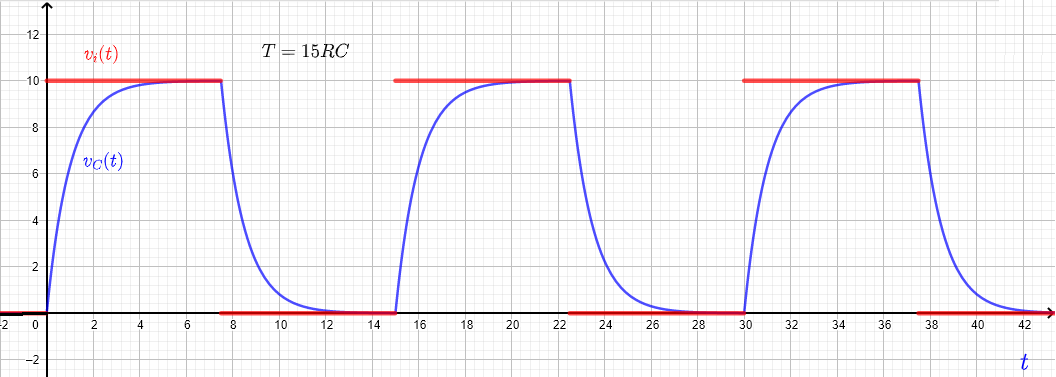Fig.3 - Graphs the input square wave and the voltage v_C(t) across the capacitor for a period T = 15 RC
b) $T = 10 RC = 10$ s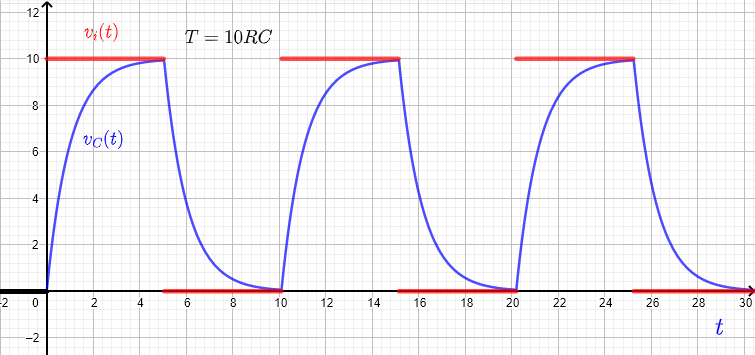Fig.4 - Graphs the input square wave and the voltage v_C(t) across the capacitor for a period T = 10 RC
c) $T = 5 RC = 5$ s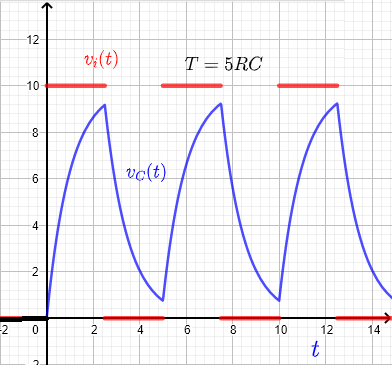Fig.5 - Graphs the input square wave and the voltage v_C(t) across the capacitor for a period T = 5 RC
d) $T = 2 RC = 2$ s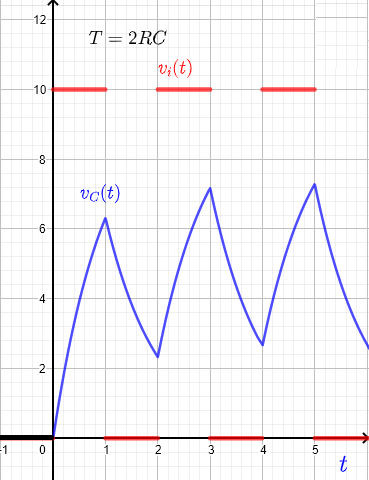Fig.6 - Graphs the input square wave and the voltage v_C(t) across the capacitor for a period T = 2 RC

## More References and Links

RC Circuit Response to a Step Voltage
Solve Differential Equations Using Laplace Transform
Laplace transforms
Engineering Mathematics with Examples and Solutions